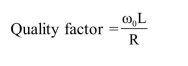# For an RLC circuit driven with voltage of amplitude

Question:

For an RLC circuit driven with voltage of amplitude $\mathrm{v}_{\mathrm{m}}$ and frequency $\mathrm{w}_{0}=\frac{1}{\sqrt{\mathrm{LC}}}$ the current exhibits resonance. The quality factor, $Q$ is given by :

1.  $\frac{\omega_{0} R}{L}$

2. $\frac{\mathrm{R}}{\left(\omega_{0} \mathrm{C}\right)}$

3. $\frac{\mathrm{CR}}{\omega_{0}}$

4.  $\frac{\omega_{0} L}{R}$

Correct Option: , 4

Solution: